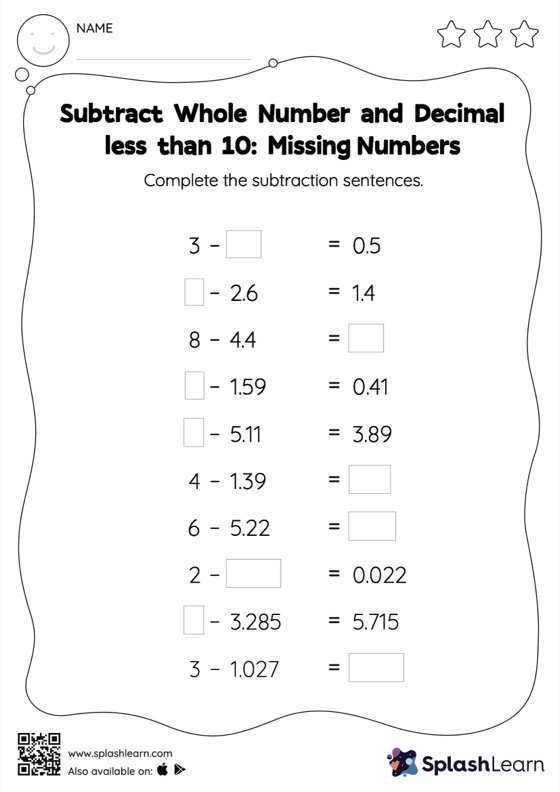# Subtract Whole Number and Decimal less than 10: Missing Numbers Worksheet

Home > Subtract Whole Number and Decimal less than 10: Missing NumbersStudents demonstrate their problem-solving ability as they work on this subtract whole number and decimal less than 10 worksheet. When subtracting decimals on this worksheet, students align the decimal points and use zero as a placeholder. Then to find the missing number in the subtract whole number and decimal less than 10 worksheet, students then apply the relationship between addition and subtraction.